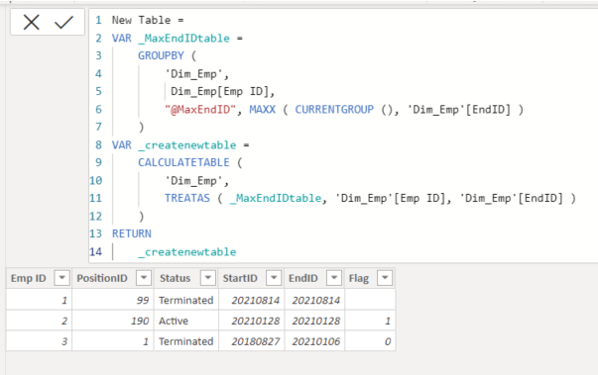cancel
Showing results for
Did you mean:Super User

## Help with Calculated Table

Hello,

I have the following DIM_Emp table:

 Emp ID PositionID Status StartID EndID Flag 1 99 Active 20191122 20210106 0 1 99 Terminated 20210814 20210814 1 78 Active 20180827 20191121 0 2 190 Active 20201029 20210106 1 2 150 Active 20200711 20201022 0 2 190 Active 20210128 20210128 1 2 12 Active 20180827 20200710 1 3 1 Terminated 20180827 20210106 0

Attempting to create a CalculatedTable as follows:  For each EmpID, get the MAX of ENDID, and then give me the other 4 fields for that record.

I have tried this a number of ways and managed to confuse myself to no end.  Hoping someone can help set me straight.

Much thanks and best regards,

1 ACCEPTED SOLUTIONSuper User

Hi @rsbin

This would be probably best done in PQ but if you want it in DAX:

``````NewTable =
FILTER (
Table1,
Table1[EndID] = CALCULATE ( MAX ( Table1[EndID] ), ALLEXCEPT ( Table1, Table1[Emp ID] ) )
)
``````

This will keep the EndID in the table. If you want it out you can use SELECTCOLUMNSPlease accept the solution when done and consider giving a thumbs up if posts are helpful.  Contact me privately for support with any larger-scale BI needs, tutoring, etc.

5 REPLIES 5Super User

Adding the SELECTCOUMNS part is simple. Here is an example for selecting two columns. If you want more, just apply the same pattern.  Check out the syntax here https://dax.guide/selectcolumns/

``````NewTable =
VAR aux_ =
FILTER (
Table1,
Table1[EndID] = CALCULATE ( MAX ( Table1[EndID] ), ALLEXCEPT ( Table1, Table1[Emp ID] ) )
)
RETURN
SELECTCOLUMNS (
aux_,
"Emp ID", Table1[Emp ID],
"PositionID", Table1[PositionID]
)``````Please accept the solution when done and consider giving a thumbs up if posts are helpful.  Contact me privately for support with any larger-scale BI needs, tutoring, etc.Super User

Hi @rsbin

This would be probably best done in PQ but if you want it in DAX:

``````NewTable =
FILTER (
Table1,
Table1[EndID] = CALCULATE ( MAX ( Table1[EndID] ), ALLEXCEPT ( Table1, Table1[Emp ID] ) )
)
``````

This will keep the EndID in the table. If you want it out you can use SELECTCOLUMNSPlease accept the solution when done and consider giving a thumbs up if posts are helpful.  Contact me privately for support with any larger-scale BI needs, tutoring, etc.Super User

@AlB,

Working on modifying your proposed solution.  There are other columns in the table that I need to disregard.  Can you guide me as to how I would modify with SELECTCOLUMNS so that I grab only the columns that I need?

Edit:  I think I figured out SELECTCOLUMNS:

=SELECTCOLUMNS(
FILTER(.....),

"column1", "column2," ... )

Thanks again and Kudos.Super User

Thank you both for the responses.  I am working in an SSAS model, so I cannot use PQ - need it in DAX.

I was attempting Jihwan's solution, but it looks like TREATAS is not a valid function.

I am now attempting AIB's solution - looks to be much simpler.

Thanks kindly to you both.  Will let you know how it works out.Super User

Hi,

Please try to write the measure something like below for creating a new table.New Table =
VAR _MaxEndIDtable =
GROUPBY (
'Dim_Emp',
Dim_Emp[Emp ID],
"@MaxEndID", MAXX ( CURRENTGROUP (), 'Dim_Emp'[EndID] )
)
VAR _createnewtable =
CALCULATETABLE (
'Dim_Emp',
TREATAS ( _MaxEndIDtable, 'Dim_Emp'[Emp ID], 'Dim_Emp'[EndID] )
)
RETURN
_createnewtable

If this post helps, then please consider accepting it as the solution to help other members find it faster, and give a big thumbs up.